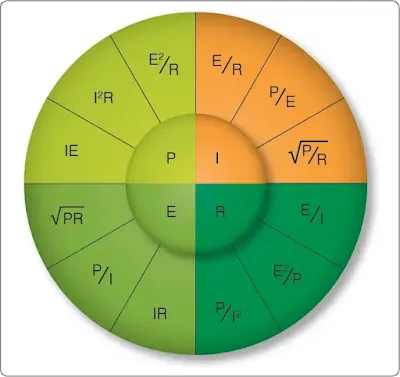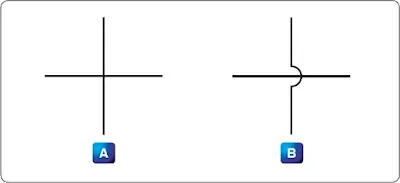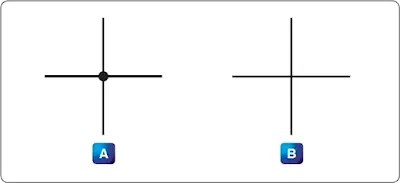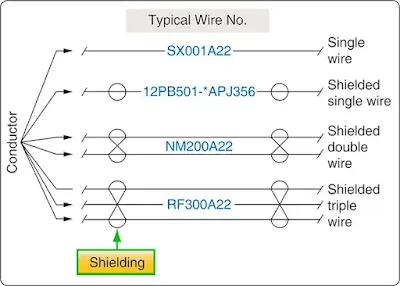# Power and Energy - Fundamentals of Electricity

## Power in an Electrical Circuit

This section covers power in the DC circuit and energy consumption. Whether referring to mechanical or electrical systems, power is defined as the rate of energy consumption or conversion within that system—that is, the amount of energy used or converted in a given amount of time.

From the scientific discipline of physics, the fundamental expression for power is:

Where
P = power measured in watts (W)
E = energy (E is a script E) measured in joules (J)
and
t = time measured in seconds (s)

The unit measurement for power is the watt (W), which refers to a rate of energy conversion of 1 joule (J)/second. Therefore, the number of joules consumed in 1 second is equal to the number of watts. A simple example is given below.

Suppose 300 joules of energy is consumed in 10 seconds. What would be the power in watts?

The watt is named for James Watt, the inventor of the steam engine. Watt devised an experiment to measure the power of a horse in order to find a means of measuring the mechanical power of his steam engine. One horsepower is required to move 33,000 pounds 1 foot in 1 minute. Since power is the rate of doing work, it is equivalent to the work divided by time. Stated as a formula, this is:

Electrical power can be rated in a similar manner. For example, an electric motor rated as a 1 horsepower motor requires 746 watts of electrical energy.

## Power Formulas Used in the Study of Electricity

When current flows through a resistive circuit, energy is dissipated in the form of heat. Recall that voltage can be expressed in the terms of energy and charge as given in the expression:

Where
E = potential difference in volts
E = energy expanded or absorbed in joules (J)
Q = charge measured in coulombs

Current I, can also be expressed in terms of charge and time as given by the expression:

Where:
I = current in amperes (A)
Q = charge in coulombs (C)
T = time

When voltage WQ  and current Qt  are multiplied, the charge Q is divided out leaving the basic expression from physics:

For a simple DC electrical system, power dissipation can then be given by the equation:

General Power Formula  P = I (E)

Where
P = Power
I = Current
E = Volts

If a circuit has a known voltage of 24 volts and a current of 2 amps, then the power in the circuit is:

P = I (E)
P = 2A (24V)
P = 48W

Now recall Ohm’s Laws that state E = I(R). If we now substitute IR for E in the general formula, we get a formula that uses only current I and resistance R to determine the power in a circuit.

P = I (IR)

Second Form of Power Equation

If a circuit has a known current of 2 amps and a resistance of 100 Ω, then the power in the circuit is:

Using Ohm’s Law, which can be stated as I = ER, we can again make a substitution such that power can be determined by knowing only the voltage (E) and resistance (R) of the circuit.

Third Form of Power Equation

If a circuit has a known voltage of 24 volts and a resistance of 20 Ω, then the power in the circuit is:

## Power in a Series and Parallel Circuit

The total power dissipated in both a series and parallel circuit is equal to the sum of the power dissipated in each resistor in the circuit. Power is simply additive and can be stated as:

Figure 1 provides a summary of all the possible transpositions of the Ohm’s Law formula and the power formula.Figure 1. Ohm’s Law formula

## Energy in an Electrical Circuit

Energy is defined as the ability to do work. Because power is the rate of energy usage, power used over a span of time is actually energy consumption. If power and time are multiplied together, we get energy.

The joule is defined as a unit of energy. There is another unit of measure which is perhaps more familiar. Because power is expressed in watts and time in seconds, a unit of energy can be called a watt-second (Ws) or more recognizable from the electric bill, a kilowatt-hour (kWh). Refer to Physics section, for further discussion on energy.

## Sources of Electricity

Electrical energy can be produced in a number of methods. The four most common are pressure, chemical, thermal, and light.

### Pressure Source

This form of electrical generation is commonly known as piezoelectric (piezo or piez taken from Greek: to press; pressure; to squeeze) is a result of the application of mechanical pressure on a dielectric or non-conducting crystal. The most common piezoelectric materials used today are crystalline quartz and Rochelle salt. However, Rochelle salt is being superseded by other materials, such as barium titanate.

The application of a mechanical stress produces an electric polarization, which is proportional to this stress. This polarization establishes a voltage across the crystal. If a circuit is connected across the crystal, a flow of current can be observed when the crystal is loaded (pressure is applied). An opposite condition can occur, where an application of a voltage between certain faces of the crystal can produce a mechanical distortion. This effect is commonly referred to as the piezoelectric effect.

Piezoelectric materials are used extensively in transducers for converting a mechanical strain into an electrical signal. Such devices include microphones, phonograph pickups, and vibration-sensing elements. The opposite effect, in which a mechanical output is derived from an electrical signal input, is also widely used in headphones and loudspeakers.

### Chemical Source

Chemical energy can be converted into electricity; the most common form of this is the battery. A primary battery produces electricity using two different metals in a chemical solution like alkaline electrolyte, where a chemical reaction between the metals and the chemicals frees more electrons in one metal than in the other. One terminal of the battery is attached to one of the metals, such as zinc; the other terminal is attached to the other metal, such as manganese oxide. The end that frees more electrons develops a positive charge and the other end develops a negative charge. If a wire is attached from one end of the battery to the other, electrons flow through the wire to balance the electrical charge.

### Thermal Sources

The most common source of thermal electricity found in the aviation industry comes from thermocouples. Thermocouples are widely used as temperature sensors. They are cheap and interchangeable, have standard connectors, and can measure a wide range of temperatures. Thermocouples are pairs of dissimilar metal wires joined at least at one end, which generate a voltage between the two wires that is proportional to the temperature at the junction. This is called the Seebeck effect, in honor of Thomas Seebeck who first noticed the phenomena in 1821. It was also noticed that different metal combinations have a different voltage difference. Thermocouples are used to measure cylinder head temperatures and Turbine Inlet Temperature (TIT).

### Light Sources

A solar cell or a photovoltaic cell is a device that converts light energy into electricity. Fundamentally, the device contains certain chemical elements that, when exposed to light energy, release electrons.

Photons in sunlight are taken in by the solar panel or cell, where they are absorbed by semiconducting materials, such as silicon. Electrons in the cell are broken loose from their atoms, allowing them to flow through the material to produce electricity. The complementary positive charges that are also created are called holes (absence of electron) and flow in the direction opposite of the electrons in a silicon solar panel.

Solar cells have many applications and have historically been used in earth orbiting satellites or space probes, handheld calculators, and wrist watches.

## Schematic Representation of Electrical Components

The schematic is the most common place where the technician finds electronic symbols. The schematic is a diagram that depicts the interconnection and logic of an electronic or electrical circuit. Many symbols are employed for use in the schematic drawings, blueprints, and illustrations. This section briefly outlines some of the more common symbols and explains how to interpret them.

### Conductors

The schematic depiction of a conductor is simple enough. This is generally shown as a solid line. However, the line types may vary depending on who drew the schematics and what exactly the line represents. While the solid line is used to depict the wire or conductor, schematics used for aircraft modifications can also use other line types, such as a dashed to represent “existing” wires prior to modification and solid lines for “new” wires.

There are two methods employed to show wire crossovers and wire connections. Figure 2 shows the two methods of drawing wires that cross: version A and version B.Figure 2. Unconnected crossover wires

Figure 3 shows the two methods for drawing wire that connect version A and version B.Figure 3. Connected wires

If version A in Figure 2 is used to depict crossovers, then version A for wire connections in Figure 3 is used. The same can then be said about the use of version B methods. The technician encounters both in common use.

Figure 4 shows a few examples of the more common wire types that the technician encounters in schematics. They are the single wire, single shielded, shielded twisted pair or double, and the shielded triple. This is not an exhaustive list of wire types but a fair representation of how they are depicted.Figure 4. Common wire types

Figure 4 also shows the wires having a wire number. These are shown for the sake of illustration and vary from one installation agency to another. For further understanding of a wire numbering system, consult the appropriate wiring guide published by the agency that drew the prints. Regardless of the specifics of the wire numbering system, organizations that exercise professional wiring practices have the installed wire marked in some manner. This is an aid to the technician who has to troubleshoot or modify the system at a later date.

RELATED POSTS